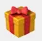# MT4: Moving Average with Stochastic Expert Advisor and how to access it by code?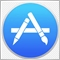35441

Hello

I would like to know how to add the moving average(s) into a separate window (not by code but manually)

the screenshot below is for that MA I am asking for: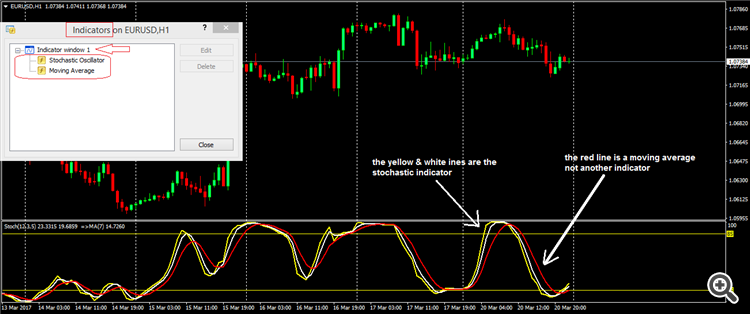..

set file attached for the above screenshot

Files: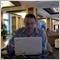Moderator
121501

Move Moving Averages indicator to separate window by mouse, select 'Apply to First Indicator's data' (see image below):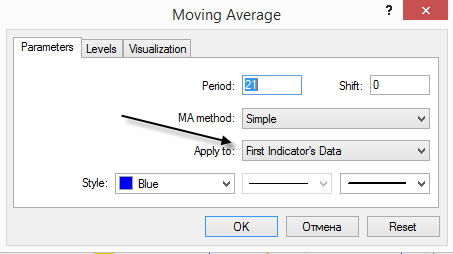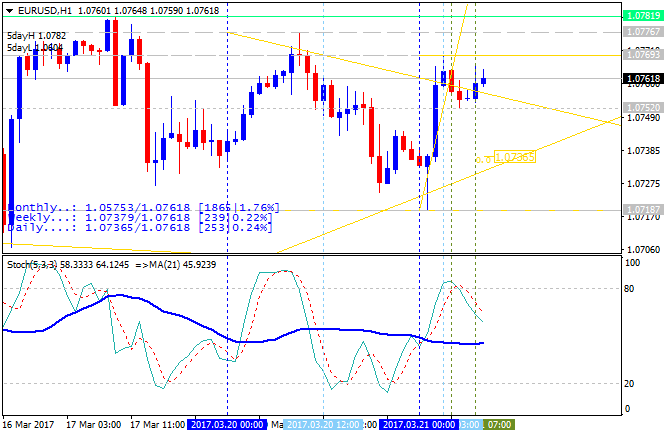But it may be the issue with the scale for stoch and for ma (scales are different) so if you will program something so it ill not be so attractive as on the images with inserting indicators manually to separate window.Moderator
121501

Template is attached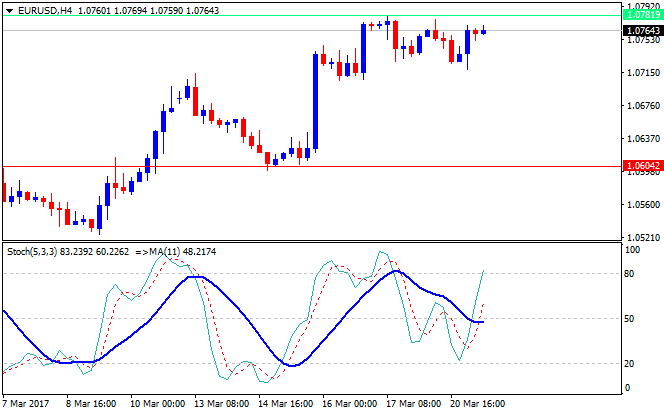Files:
mastoch.tpl  2 kb35441

Thank You Sergey

Good ...
Remaining how to access that Stoch Moving Average in building experts (EAs)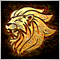Moderator
13500

You pass it as a parameter.

```iMA

The function returns the handle of the Moving Average indicator. It has only one buffer.

int  iMA(
string               symbol,            // symbol name
ENUM_TIMEFRAMES      period,            // period
int                  ma_period,         // averaging period
int                  ma_shift,          // horizontal shift
ENUM_MA_METHOD       ma_method,         // smoothing type
ENUM_APPLIED_PRICE   applied_price      // type of price or handle
);

```

Parameters

symbol

[in] The symbol name of the security, the data of which should be used to calculate the indicator. The NULL value means the current symbol.

period

[in] The value of the period can be one of the ENUM_TIMEFRAMES values, 0 means the current timeframe.

ma_period

[in] Averaging period for the calculation of the moving average.

ma_shift

[in]  Shift of the indicator relative to the price chart.

ma_method

[in]  Smoothing type. Can be one of the ENUM_MA_METHOD values.

applied_price

[in]  The price used. Can be any of the price constants ENUM_APPLIED_PRICE or a handle of another indicator.

Return Value

Returns the handle of a specified technical indicator,  in case of failure returns INVALID_HANDLE. The computer memory can be freed from an indicator that is no more utilized, using the IndicatorRelease() function, to which the indicator handle is passed.35441

Marco vd Heijden:

You pass it as a parameter.

Parameters

symbol

[in] The symbol name of the security, the data of which should be used to calculate the indicator. The NULL value means the current symbol.

period

[in] The value of the period can be one of the ENUM_TIMEFRAMES values, 0 means the current timeframe.

ma_period

[in] Averaging period for the calculation of the moving average.

ma_shift

[in]  Shift of the indicator relative to the price chart.

ma_method

[in]  Smoothing type. Can be one of the ENUM_MA_METHOD values.

applied_price

[in]  The price used. Can be any of the price constants ENUM_APPLIED_PRICE or a handle of another indicator.

Return Value

Returns the handle of a specified technical indicator,  in case of failure returns INVALID_HANDLE. The computer memory can be freed from an indicator that is no more utilized, using the IndicatorRelease() function, to which the indicator handle is passed.

Thanks Marco!

That is the standard..
That is only for on-chart moving average
But how to call it in our case?

In another style, how to link it to the stochastic?Moderator
13500

Pass it the stochastic handle.

The yellow part.

applied_price

[in]  The price used. Can be any of the price constants ENUM_APPLIED_PRICE or a handle of another indicator.35441

Marco vd Heijden:

You pass it as a parameter.

```iMA

The function returns the handle of the Moving Average indicator. It has only one buffer.

int  iMA(
string               symbol,            // symbol name
ENUM_TIMEFRAMES      period,            // period
int                  ma_period,         // averaging period
int                  ma_shift,          // horizontal shift
ENUM_MA_METHOD       ma_method,         // smoothing type
ENUM_APPLIED_PRICE   applied_price      // type of price or handle
);
```

Parameters

symbol

[in] The symbol name of the security, the data of which should be used to calculate the indicator. The NULL value means the current symbol.

.

.

.

.

Hey Marco,

Finally I found it

the trick is not in the

`iMA(....);`

it is the:

```iMAOnArray();
```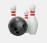```iMAOnArray

Calculates the Moving Average indicator on data, stored in array, and returns its value.

double  iMAOnArray(
double       array[],          // array with data
int          total,            // number of elements
int          ma_period,        // MA averaging period
int          ma_shift,         // MA shift
int          ma_method,        // MA averaging method
int          shift             // shift
);

Parameters

array[]

[in]  Array with data.

total

[in]  The number of items to be counted. 0 means the whole array.

ma_period

[in]  Averaging period for calculation.

ma_shift

[in]  MA shift. Indicators line offset relate to the chart by timeframe.

ma_method

[in]  Moving Average method. It can be any of ENUM_MA_METHOD enumeration values.

shift

[in]  Index of the value taken from the indicator buffer (shift relative to the current bar the given amount of periods ago).

Returned value

Numerical value of the Moving Average indicator, calculated on data, stored in array[].

Note

Unlike iMA(...), the iMAOnArray() function does not take data by symbol name, timeframe, the applied price. The price data must be previously prepared. The indicator is calculated from left to right. To access to the array elements as to a series array (i.e., from right to left), one has to use the ArraySetAsSeries() function.

Example:

double macurrent=iMAOnArray(ExtBuffer,0,5,0,MODE_LWMA,0);
double macurrentslow=iMAOnArray(ExtBuffer,0,10,0,MODE_LWMA,0);
double maprev=iMAOnArray(ExtBuffer,0,5,0,MODE_LWMA,1);
double maprevslow=iMAOnArray(ExtBuffer,0,10,0,MODE_LWMA,1);
//----
if(maprev<maprevslow && macurrent>=macurrentslow)
Alert("crossing up");

```

Thanks for all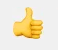Moderator
29969

Mohammad Soubra:

Hey Marco,

Finally I found it

the trick is not in the

iMA(....);

it is the:

```iMAOnArray();
```

Thanks for all

That is the answer that I gave you in your other topic

https://www.mql5.com/en/forum/172819#comment_4198063MOVING AVERAGES IN SEPARATE INDICATOR WINDOW
• www.mql5.com
Hello may I know how to add the moving averages to a separate window as below screenshot...35441

Keith Watford:

That is the answer that I gave you in your other topic

https://www.mql5.com/en/forum/172819#comment_4198063

oh...

yes

seems I haven't detect it between the lines

thanks for you

appreciated